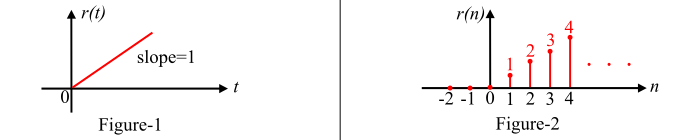# What is a Unit Ramp Signal?

A signal is defined as a single-valued function of one or more independent variables which contain some information. Examples of signals are electric current and voltage, human speech, etc.

## Unit Ramp Signal

A ramp function or ramp signal is a type of standard signal which starts at 𝑡 = 0 and increases linearly with time. The unit ramp function has unit slop.

## Continuous-Time Unit Ramp Signal

The continuous-time unit ramp signal is that function which starts at 𝑡 = 0 and increases linearly with time. It is denoted by r(t). Mathematically, the continuous-time unit ramp signal is defined as follows −

$$\mathrm{r(t)=\left\{\begin{matrix} 1\; \; for\; t\geq 0\ 0\; \; for\: t< 0\ \end{matrix}\right.}$$

Also,

𝑟(𝑡) = 𝑡 𝑢(𝑡)

From the above equation, it is clear that the ramp signal is a signal whose magnitude varies linearly. The graphical representation of the continuous-time unit ramp signal is shown in Figure-1.## Discrete-Time Unit Ramp Sequence

The discrete time unit ramp signal is that function which starts from n = 0 and increases linearly. It is denoted by r(n). It is signal whose amplitude varies linearly with time n. mathematically, the discrete time unit ramp sequence is defined as −

$$\mathrm{r(n)=\left\{\begin{matrix} n\; \; for\; n\geq 0\ 0\; \; for\: n< 0\ \end{matrix}\right.}$$

Or,

𝑟(𝑛) = 𝑛 𝑢(𝑛)

The graphical representation of a discrete-time unit ramp sequence is shown in Figure-2.

## Relationship between Unit Ramp and Unit Step Signals

The unit ramp signal can be obtained by integrating the unit step signal with respect to time. In other words, a unit step signal can be obtained by differentiating the unit ramp signal.

The unit step signal is given by,

$$\mathrm{u(t)=\left\{\begin{matrix} 1\; \; for\; t\geq 0\ 0\; \; for\: t< 0\ \end{matrix}\right.}$$

Therefore, the unit ramp function is,

$$\mathrm{r(t)=\int\: u(t)dt=\int dt=t;\; \; \; for\: t\geq 0 }$$

Also,

$$\mathrm{u(t)=\frac{\mathrm{d} }{\mathrm{d} t}r(t)}$$